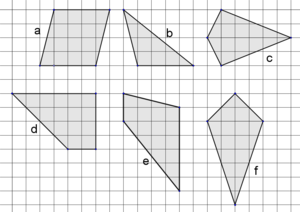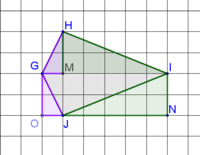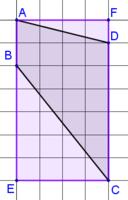Engage your students with effective distance learning resources. ACCESS RESOURCES>>

Alignments to Content Standards: 6.G.A.1

Of the polygons shown, which have equal areas? Explain how you know.IM Commentary

The purpose of this task is for students to use what they know about area to find the areas of special quadrilaterals. Depending on previous instruction, methods may includeÂ decomposingÂ the figuresÂ into right triangles and rectangles, orÂ drawing a rectangle to encircle the figure and subtracting areas of right triangles that are not part of the original figure. Students may also make use of area formulas that they have previously derived, like $A=bh/2$ for triangles or $A = bh$ for parallelograms. The standards do notÂ expect studentsÂ to know or use a formula for the area of a trapezoid or area of a kite, though a teacher could use this task as part of a lesson on generating those formulas if so desired.Â

An open-ended task like this provides a great opportunity forÂ students to explain their reasoning and may lead to studentÂ critiques of each other's reasoning MP.3. As students work, teachers should be on the lookout for alternate approaches. Students could be asked to share their approach with the class, or different approaches could be highlighted in something like a gallery walk.Â

For students who finish quickly, anÂ extension question of slightly higher difficulty might be to ask students to draw additional quadrilaterals with area 24 that are neitherÂ rectangles nor parallelograms, or additional polygons with area 24 that include no right angles.

Solution

Polygons a, d, e, and fÂ eachÂ hasÂ an area of 16 square units.

(The area of polygon b is 8 square units, and the area of polygon c is 12 square units.)

Depending on previousÂ instruction, methods may includeÂ decomposing/composing the figuresÂ into rightÂ triangles and rectangles [see (1) below], orÂ drawing a rectangle to encircle the figure and subtracting areas of right triangles that are not part of the original figure [see (2) below]. Students may also make use of area formulas that they have previously derived, like A=bh/2 for triangles or A = bh for parallelograms.

(1) The figure below shows polygon c, kite HGJI. Right triangle HGM is relocated to right triangle GJO, and right triangle MIH is relocated to right triangle NJI. This creates rectangle GONI, which has the same area as the original kite, 2 by 6 = 12 square units.(2) The figure below shows polygon e with rectangle AFCEÂ superimposed. If we start with the area of rectangle AFCE (4 by 7 = 28 square units), subtract the areas of triangles AFD (2 square units) and BEC (10 square units), we deduce that the area of polygonÂ e is 28 - 2 - 10 = 16 square units.Instead of calculating the area of each figure, students may instead compareÂ or overlayÂ congruent components of figures. This is a great approach, and discussion could encourage all studentsÂ to understand several ways to approach the same problem. For example, the figure below shows that polygon c has a smaller area than polygon f.